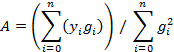Non-Linear Curve Fits

INTRODUCTION

Frequently we have a table of values as a function of a single variable which may have measurement noise or other inaccuracies.   These would be of the form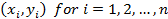What we want to do then is to find the “m” parameters “λ” of some function f(x; λ) which is “non-linear” in the parameters and provides the best fit to the tabulated data.

NON-LINEAR FITS

Given some function, then at each data point we can write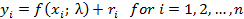for the “m” parameters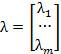and where “” represents the uncertainty or measurement error  as a consequence of an imperfect initial estimate of the parameters.   We can then approximate the errors as a linear combination of corrections to our parameter set aswherewhich could be used to iteratively update the original set as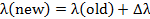Writing out the entire matrix we get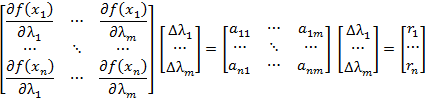or more conciselyBut this is an over determined set of equations and not in form allowing for a unique determination of the parameter corrections,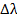.   So we multiply each side by the transpose of the matrix “A” which switches rows and columns as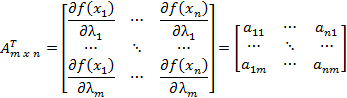So thatwhereand for each element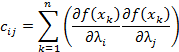And also by recalling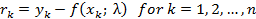then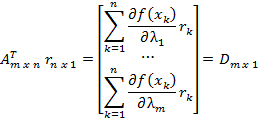or finally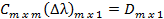which allows for a unique solution for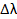values.

GAUSSIAN FUNCTION

The well known Gaussian distribution (also called the “Normal” distribution or Bell curve) has the general functional formwhere the three parameters are given by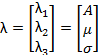In this parameter set, A is area under the curve of G(x).  The arithmetic average or mean is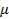.   And the last parameter σ is the standard distribution.   These are further described in the Appendix: Gaussian Parameters.

We can write the derivatives of the Gaussian with respect to its three parameters as follows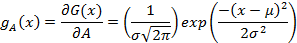The iterative corrections to the initial guess for the three parameters can be expressed in the equationor in detail, this expands to the followingFor the measurements of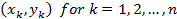then the individual matrix coefficients are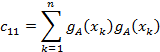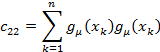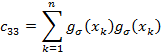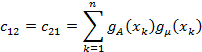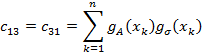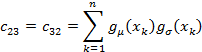And definingthen the matrix elements are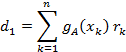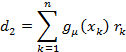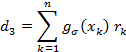We could solve the matrix equation using Gauss-Jordan but for only three parameters it is easier to write the solutions directly as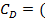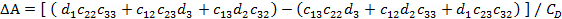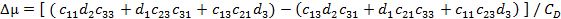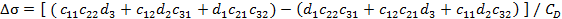And this allows us to iteratively update the initial guesses for the parameters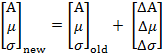APPENDIX: GAUSSIAN PARAMETERS

For a random variable, X, perhaps with a Gaussian probability distribution, the results of “n” independent measurements can be represented as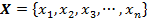The arithmetic average or mean of this series of “n” values is simply given by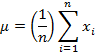and the variance (or square of the standard distribution) for a large number of “n” trials can be written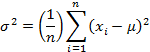These equations are exact and work well for a small number of samples.  But as the number of samples increase, it is easier to create a histogram.

Basically the data is grouped into a set of “m” bins where “yj” represents the number of values which lie within some small range, “Δx”, around a midpoint of “xj”.  Each small range represents a separate bin and can be drawn as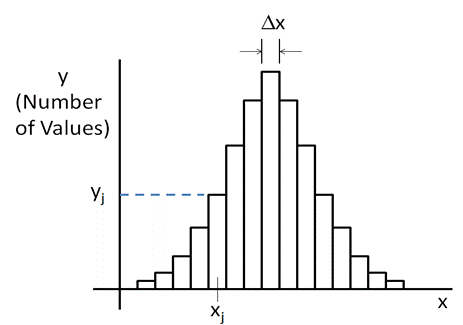As before the expression for the mean is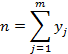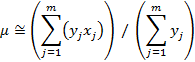and the variance can be written as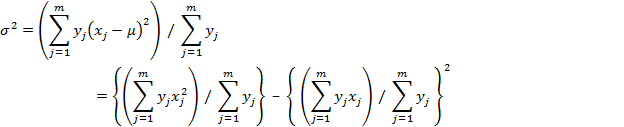As the number of discrete bins increases and the width “Δx” of each gets smaller, then the bins come to resemble a continuous curve as follows: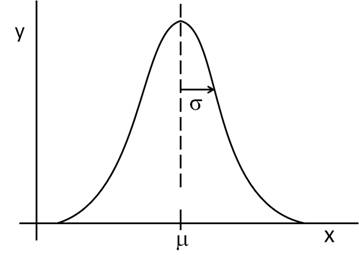For a continuous curve the area under the Gaussian is given by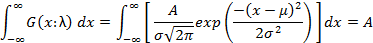The mean value can then be written as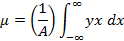And the variance is given by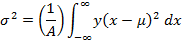APPENDIX: INITIAL ESTIMATE

For a discrete set of data pointsthen if we first define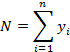then the arithmetic average or mean value of the distribution is given byand the variance or square of the standard deviation is given by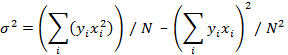But since we are linear in A, we can write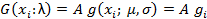where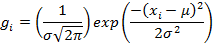then the total error associated with an initial guess for A is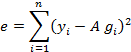and we can minimize A in a least squares sense by setting the derivative to zero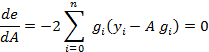or